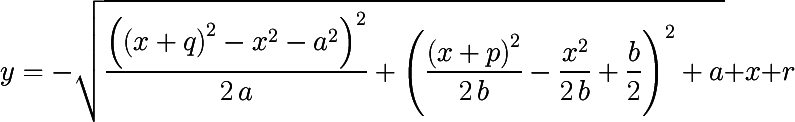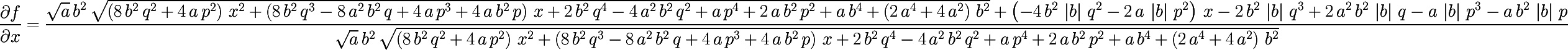# Jayduino

Arduino stuff written by me and released into the public domain.
To Be Published:
• LCD64240B a huge matrix display (240x64)
• VFD128x64 another nice VFD matrix display (128x64 pixels)

## BMA180

Library (not complete) for BOSCH BMA180 acceleration sensor. Note that you need to convert the levels of the sensor to 3.3V. Comunication via I2C interface. There is also an example to use the Interrupt pin of the BMA180.

## DS1307 RTC

Library for the Real Time Clock DS1307. Contains also interrupt driven example for the SQW pin.
Download the library: DS1307.zip or browse the files. Some tips: if the DS1306 SQW/OUT deos not work even if you have set the register and you are sure that your ISR is working you need to:
• switch off everything
• unplug the backup battery
• switch on
• plug the battery (on or off, doesn't matter)
• Reset the time and activate the ctrl_register
This should bring it back to life. If you don't want a battery backup: connect the Battery VDD to GND. I personally use the DS1307 mostly for realtime logging and clocks and the ISR for accurate pitch detection (the arduino is sometimes badly off). For the pitch detection i use the 32Khz interrupt and a counter. for every second should be exactely 32768 ticks (depends how much the 32Khz OSC is off).

## DCF77 library

DCF77 is an RF sender that transmits timecodes in central europe, using a simple receiver you can retrieve the exact time.
My DCF77 library contains various useful things like:
• signal quality indication
• minimum use of sampling (using external interrupt pin 2 or 3)
• minute and seconds callback functions
• plausibility check on data
• drift calculation of internal clock (msecs/min)
Included are various examples showing it's use in combination with:
• serial mode
• a real time clock (you need the DS1307 library)
• vfd-displays + RTC
• servos (dynamixel AX-12) as a watch
but be warned, it is far from complete and clean.

A way to read the analog input with less waitstates (make a sampling request, do what you like to do, read the value). Also to make the acquisition faster (but less precise resolution).

## VFDM12

A library for the nice (and cheap) 12 digits flourescent display Futaba VFD M12BY022AA. Comunication via I2C (so you leave your Serial port free and it is damn fast).

## ysecTimer2

Library that 'hijacks' the Timer2 with ysecs resolution. Ideal for some kinds of data acquisition (> 1khz).

## Filter Suite

A form to create realtime filters (i.e. audio or for nervous sensors). Optimized for Arduino short to long (16bit signed) operations. Filters included are
All filters use constant coefficients to obtain faster calculation (so no dynamic filters included). you have only to calculate the factor of your filter (hz/samplerate) and include the filter you need. I am counting on adding convolution and pitchdetection algorithms and to provide a web interface so you can design your own DIY ready-to-use filter code.

## Newton method for sound distance analysis (TDOA)

Not actually so much Arduino, but I needed this to solve a problem for an automatic remote 3D-helicopter camera: find the EXACT position of an heli using four mics placed at corners of a rectangle. The heli emits an ultrasonic sound pattern (kind of inversed bat). I think it could have applications in many situations. Measuring positions of any noise creating object. Helping blind people orientation. Active precise measurement of positions in athletics (with an ultrasonic emitter).
New: Gave a try to matlab to solve the equation and it got a result, BUT when testing them they did not get the right results!
```>> solve('r+t-sqrt((a-((q+t)^2-t^2-a^2)/(-2*a))^2+(b-((p+t)^2-t^2-b^2)/(-2*b))^2)')
t=-(a^2*p^3 + b^2*q^3 + a^2*b^2*p + a^2*b^2*q - 2*a^2*b^2*r + a*b*(a^4*b^2 - a^4*p^2 + a^2*b^4 + 2*a^2*b^2*p^2 + 2*a^2*b^2*p*q - 4*a^2*b^2*p*r + 2*a^2*b^2*q^2 - 4*a^2*b^2*q*r + a^2*p^4 + 2*a^2*p^3*q - 4*a^2*p^3*r - 2*a^2*p^2*q^2 + 4*a^2*p^2*r^2 - b^4*q^2 - 2*b^2*p^2*q^2 + 2*b^2*p*q^3 + b^2*q^4 - 4*b^2*q^3*r + 4*b^2*q^2*r^2 - p^4*q^2 + 2*p^3*q^3 - p^2*q^4)^(1/2))/(- 2*a^2*b^2 + 2*a^2*p^2 + 2*b^2*q^2)
t=-(a^2*p^3 + b^2*q^3 + a^2*b^2*p + a^2*b^2*q - 2*a^2*b^2*r - a*b*(a^4*b^2 - a^4*p^2 + a^2*b^4 + 2*a^2*b^2*p^2 + 2*a^2*b^2*p*q - 4*a^2*b^2*p*r + 2*a^2*b^2*q^2 - 4*a^2*b^2*q*r + a^2*p^4 + 2*a^2*p^3*q - 4*a^2*p^3*r - 2*a^2*p^2*q^2 + 4*a^2*p^2*r^2 - b^4*q^2 - 2*b^2*p^2*q^2 + 2*b^2*p*q^3 + b^2*q^4 - 4*b^2*q^3*r + 4*b^2*q^2*r^2 - p^4*q^2 + 2*p^3*q^3 - p^2*q^4)^(1/2))/(- 2*a^2*b^2 + 2*a^2*p^2 + 2*b^2*q^2)
```

### the working program

```
double a=210; // distance in m
double b=140; // distance in m

#define SQR(x) ((x)*(x))
#define TRI(x) ((x)*(x)*(x))

/* and this are the functions f(x) and f'(x) for the newton method
* approximation step is xn1=xn-f(xn, p, q, r)/f1(xn, p, q, r);
***/

double f(double t, double p, double q, double r)
{
return r+t-sqrt(SQR(a-(SQR(q+t)-SQR(t)-SQR(a))/(-2*a))+SQR(b-(SQR(p+t)-SQR(t)-SQR(b))/(-2*b)));
}

/* ok, this one is terrible... ;)
*/
double f1(double x, double p, double q, double r)
{
return (SQR(a)*SQR(b)*sqrt((4*SQR(b)*SQR(q)+4*SQR(a)*SQR(p))*SQR(x)+
(4*SQR(b)*TRI(q)+4*SQR(a)*SQR(b)*q+4*SQR(a)*TRI(p)+
(-2*fabs(a)*SQR(b)*fabs(b)*SQR(q)-2*SQR(a)*fabs(a)*fabs(b)*SQR(p))*x-
fabs(a)*SQR(b)*fabs(b)*TRI(q)-SQR(a)*fabs(a)*SQR(b)*fabs(b)*q-
SQR(a)*fabs(a)*fabs(b)*TRI(p)-SQR(a)*fabs(a)*SQR(b)*fabs(b)*p)/
(SQR(a)*SQR(b)*sqrt((4*SQR(b)*SQR(q)+4*SQR(a)*SQR(p))*SQR(x)+
(4*SQR(b)*TRI(q)+4*SQR(a)*SQR(b)*q+4*SQR(a)*TRI(p)+4*SQR(a)*SQR(b)*p)*x+
}

void getxy(double t, double p, double q, double a, double b, double *x, double *y)
{
*x=(SQR(q)-SQR(t)-SQR(a))/(-2*a);
*y=(SQR(p)-SQR(t)-SQR(b))/(-2*b);
}

/* pass the measured times of a qudrant delta t's, t3 being the opposite corner of the qudrant.
*/
void newtonFindXY(double t1, double t2, double t3, double eps, double *xres, double *yres)
{
double xn1;
double xn=(a+b)/2-t3;

printf("x=%.1f ",xn);
for (int i=1; i <= 10; i++)
{
xn1=xn-f(xn, t1, t2, t3)/f1(xn, t1, t2, t3);
printf("%.1f ",xn1);
if (fabs(xn-xn1) < eps)
{
break;
}
xn=xn1;
}
getxy(xn1, t1+xn1, t2+xn1, a, b, xres, yres);
}
```

Enjoy or send an email to Jürgen Schwietering